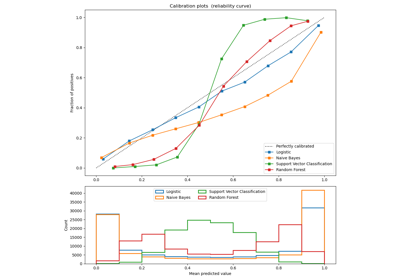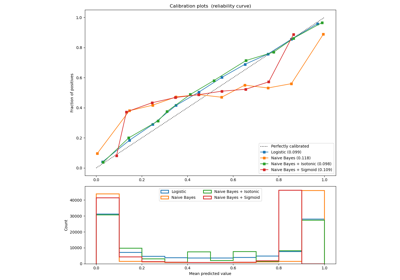# sklearn.calibration.calibration_curve¶

sklearn.calibration.calibration_curve(y_true, y_prob, *, normalize=False, n_bins=5, strategy='uniform')[source]

Compute true and predicted probabilities for a calibration curve.

The method assumes the inputs come from a binary classifier, and discretize the [0, 1] interval into bins.

Calibration curves may also be referred to as reliability diagrams.

Read more in the User Guide.

Parameters
y_truearray-like of shape (n_samples,)

True targets.

y_probarray-like of shape (n_samples,)

Probabilities of the positive class.

normalizebool, default=False

Whether y_prob needs to be normalized into the [0, 1] interval, i.e. is not a proper probability. If True, the smallest value in y_prob is linearly mapped onto 0 and the largest one onto 1.

n_binsint, default=5

Number of bins to discretize the [0, 1] interval. A bigger number requires more data. Bins with no samples (i.e. without corresponding values in y_prob) will not be returned, thus the returned arrays may have less than n_bins values.

strategy{‘uniform’, ‘quantile’}, default=’uniform’

Strategy used to define the widths of the bins.

uniform

The bins have identical widths.

quantile

The bins have the same number of samples and depend on y_prob.

Returns
prob_truendarray of shape (n_bins,) or smaller

The proportion of samples whose class is the positive class, in each bin (fraction of positives).

prob_predndarray of shape (n_bins,) or smaller

The mean predicted probability in each bin.

References

Alexandru Niculescu-Mizil and Rich Caruana (2005) Predicting Good Probabilities With Supervised Learning, in Proceedings of the 22nd International Conference on Machine Learning (ICML). See section 4 (Qualitative Analysis of Predictions).

## Examples using sklearn.calibration.calibration_curve¶